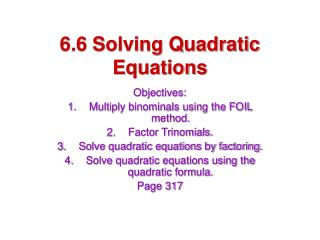DownloadDownload PresentationTélécharger la présentation- - - - - - - - - - - - - - - - - - - - - - - - - - - E N D - - - - - - - - - - - - - - - - - - - - - - - - - - -
##### Presentation Transcript

1. 6.6 Solving Quadratic Equations Objectives: Multiply binominals using the FOIL method. Factor Trinomials. Solve quadratic equations by factoring. Solve quadratic equations using the quadratic formula. Page 317

2. A binomial expression has just two terms (usually an x term and a constant). There is no equal sign.  Its general form is ax + b, where a and b are real numbers and a ≠ 0. • One way to multiply two binomials is to use the FOIL method. FOIL stands for the pairs of terms that are multiplied: First, Outside, Inside, Last. • This method works best when the two binomials are in standard form (by descending exponent, ending with the constant term). • The resulting expression usually has four terms before it is simplified. Quite often, the two middle (from the Outside and Inside) terms can be combined.

3. For example:

4. The opposite of multiplying two binomials is to factor or break down a polynomial (many termed) expression. • Several methods for factoring are given in the text. Be persistent in factoring! It is normal to try several pairs of factors, looking for the right ones. • The more you work with factoring, the easier it will be to find the correct factors. • Also, if you check your work by using the FOIL method, it is virtually impossible to get a factoring problem wrong.  • Remember!  When factoring, always take out any factor that is common to all the terms first.

5. A quadratic equation involves a single variable with exponents no higher than 2. • Its general form is where a, b, and c are real numbers and .  • For a quadratic equation it is possible to have two unique solutions, two repeated solutions (the same number twice), or no real solutions. • The solutions may be rational or irrational numbers.

6. To solve a quadratic equation, if it is factorable: •     1.  Make sure the equation is in the general form.  •     2.  Factor the equation. •     3.  Set each factor to zero.  •     4.  Solve each simple linear equation.

7. To solve a quadratic equation if you can’t factor the equation: • Make sure the equation is in the general form.  • Identify a, b, and c. • Substitute a, b, and c into the quadratic formula: • Simplify.

8. The beauty of the quadratic formula is that it works on any quadratic equation when put in the form general form. • If you are having trouble factoring a problem, the quadratic formula might be quicker. • Always be sure and check your solution in the original quadratic equation.

9. <> <>

10. Find the product:

11. Factor x2 - 7x + 12. 1. Pairs of numbers which make 12 when multiplied: (1, 12), (2, 6), and (3, 4). • 1 + 12≠7. 2 + 6≠7. 3 + 4 = 7. Thus, d = 3 and e = 4. • (x - 3)(x - 4) • Check: (x - 3)(x - 4) = x2 -4x - 3x + 12 = x2 - 7x + 12 • Thus, x2 - 7x + 12 = (x - 3)(x - 4).

12. Factor 2x3 +4x2 + 2x. First, remove common factors: 2x3 +4x2 +2x = 2x(x2 + 2x + 1) • Pairs of numbers which make 1 when multiplied: (1, 1). • 1 + 1 = 2. Thus, d = 1 and e = 1. • 2x(x + 1)(x + 1) (don't forget the common factor!) • Check: 2x(x + 1)(x + 1) = 2x(x2 +2x + 1) = 2x3 +4x2 + 2x • Thus, 2x3 +4x2 +2x = 2x(x + 1)(x + 1) = 2x(x + 1)2.x2 + 2x + 1 is a perfect square trinomial.

13. The Box Method for Factoring a Polynomial

14. The Box Method for Factoring a Polynomial

15. Factor the trinomial:

16. Use the Quadratic Formula to solve

17. Solve for x:

18. Solve for x:

19. Solve using the quadratic formula:

20. Homework Assignment on the Internet Section 6.6 (Read Solving Quadratic Equation) Pp 329-330: 2-78even.